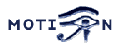Motion - Support Question 2007x 03x 01x 075407
You are here: Foswiki>Motion Web>SupportRequests>SupportQuestion2007x03x01x075407 (04 Mar 2007, AngelCarpintero)

# "location" option not working as expected

## Question

I've set "location" to "preview" and would have expected to get a rectangle drawed around the very first motion detetcted in the motion jpegs. Allthough, only "on" or "off" seems to work and "preview" has no effect. Settings:
```daemon = on
process_id_file = (not defined)
setup_mode = off
videodevice = /dev/video0
input = 0
norm = 0
frequency = 0
rotate = 0
width = 704
height = 576
framerate = 29
minimum_frame_time = 0
netcam_url = (not defined)
netcam_userpass = (not defined)
netcam_proxy = (not defined)
auto_brightness = off
brightness = 0
contrast = 0
saturation = 0
hue = 0
roundrobin_frames = 0
roundrobin_skip = 0
switchfilter = off
threshold = 900
threshold_tune = on
noise_level = 2
noise_tune = on
night_compensate = on
despeckle = el
lightswitch = 60
minimum_motion_frames = 2
pre_capture = 4
post_capture = 30
gap = 30
max_mpeg_time = 0
low_cpu = 5
output_all = off
output_normal = on
output_motion = off
quality = 100
ppm = off
ffmpeg_cap_new = on
ffmpeg_cap_motion = off
ffmpeg_timelapse = 60
ffmpeg_timelapse_mode = daily
ffmpeg_bps = 4000000
ffmpeg_variable_bitrate = 2
ffmpeg_video_codec = msmpeg4
ffmpeg_deinterlace = on
snapshot_interval = 60
locate = preview
text_right = %d.%m.%Y\n%T Frame %q\nNoise: %N, Threshold %o
text_left = Kamera 1 (Eingang)
text_changes = on
text_event = %Y%m%d%H%M%S
text_double = off
target_dir = .......WebCam
snapshot_filename = cam0/current
jpeg_filename = Events/%Y/%m/%d//%v.0/%H%M%S-%q-%D
movie_filename = Events/%Y/%m/%d//%v.0/%H%M%S-%q
timelapse_filename = Events/%Y/%m/%d/cam0-timelapse
webcam_port = 8081
webcam_quality = 75
webcam_motion = off
webcam_maxrate = 10
webcam_localhost = off
webcam_limit = 0
control_port = 8080
control_localhost = off
control_html_output = on
control_authentication = .......
track_type = 4
track_auto = off
track_port = (not defined)
track_motorx = -1
track_motory = 0
track_maxx = 0
track_maxy = 0
track_iomojo_id = 0
track_step_angle_x = 10
track_step_angle_y = 10
track_move_wait = 10
track_speed = 255
track_stepsize = 40
quiet = off
on_event_start = (not defined)
on_event_end = (not defined)
on_picture_save = (not defined)
on_motion_detected = (not defined)
on_movie_start = (not defined)
on_movie_end = (not defined)
sql_log_image = off
sql_log_snapshot = off
sql_log_mpeg = off
sql_log_timelapse = off
sql_query = sql_query insert into security(camera, filename, frame, file_type, time_stamp, event_time_stamp) values('%t', '%f', '%q', '%n', '%Y-%m-%d %T', '%C')
mysql_db = (not defined)
mysql_host = (not defined)
mysql_user = (not defined)
video_pipe = (not defined)
motion_video_pipe = (not defined)
```
Any Ideas?

```
```

## Environment

 Motion version: 3.2.8 ffmpeg version: 0.4.8 Libraries: ffmpeg Server OS: SuSE 9.2

-- AxelAmthor - 01 Mar 2007

'locate preview' only works if 'output_normal' is set to either 'first' or 'best'.

-- AndrewHamilton - 01 Mar 2007